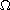## Longitudinal librations of a satellite

When
Start: 11/09/2011 - 1:15pm
End  : 11/09/2011 - 2:15pm

Category
Applied Math Seminar

Speaker
Mario Martelli, CGU

Abstract

Furi, Martelli and Landsberg gave a theoretical explanation of the chaotic longitudinal librations of Hyperion, a satellite of Saturn. The analysis was made under the simplifying assumption that the spin axis remains perpendicular to the orbit plane. Here, under the same assumption, we investigate the behavior of the longitudinal librations of any satellite. Also we show that they are possibly chaotic depending on two parameters: a constant k related to the principal moments of inertia of the satellite, and the eccentricity e of its orbit. We prove that the plane k-e contains an open regionwith the property that the longitudinal librations of any satellite are possibly chaotic if the point (k,e) belongs to this region. Since Hyperion's point is inside, the results of this paper are more general than those obtained previously.

Where
Davidson, CMC

Proudly Serving Math Community at the Claremont Colleges Since 2007
Copyright © 2018 Claremont Center for the Mathematical Sciences